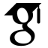Titel: Extremal problems in 3-uniform hypergraphs Sprache: Englisch Autor*in: Piga, Simón Erscheinungsdatum: 2021 Tag der mündlichen Prüfung: 2022-05-30 Zusammenfassung: In this thesis we proved three results for 3-uniform dense hypergraphs. In each case, we determined conditions for the existence of different kinds of substructures. In the first one, we show that 3-uniform hypergraphs with the property that all vertices have a quasirandom link graph with density bigger than 1/3 contain a clique on five vertices. This result is asymptotically best possible. With this, we solved an open problem left by Reiher, Rödl, and Schacht about Turán densities in uniformly dense hypergraphs. For the second problem, we study sufficient conditions for the existence of Hamilton cycles in uniformly dense 3-uniform hypergraphs. Problems of this type were first considered by Lenz, Mubayi, and Mycroft for loose Hamilton cycles and Aigner-Horev and Levy considered it for tight Hamilton cycles for a fairly strong notion of uniformly dense hypergraphs. We focus on tight cycles and obtain optimal results for a weaker notion of uniformly dense hypergraphs. We show that if an n-vertex 3-uniform hypergraph H=(V,E) has the property that for any set of vertices X and for any collection P of pairs of vertices, the number of hyperedges composed by a pair belonging to P and one vertex from X is at least (1/4+o(1))|X||P| - o(|V|^3) and H has minimum vertex degree at least \Omega(|V|^2), then H contains a tight Hamilton cycle. A probabilistic construction shows that the constant 1/4 is optimal in this context.Finally, we show that 3-uniform hypergraphs on n vertices whose codegree is at least (2/3 + o(1))n can be decomposed into tight cycles subject to the trivial necessary divisibility conditions. This result can be used to prove the existence of a tight Euler tour under the same minimum codegree condition.We provide a construction showing that our bounds are best possible up to the o(1) term. All together, our results address recent open problems by Glock, Kühn, and Osthus. URL: https://ediss.sub.uni-hamburg.de/handle/ediss/9645 URN: urn:nbn:de:gbv:18-ediss-101049 Dokumenttyp: Dissertation Betreuer*in: Schacht, Mathias Enthalten in den Sammlungen: Elektronische Dissertationen und Habilitationen

###### Dateien zu dieser Ressource:
Datei Beschreibung Prüfsumme GrößeFormat

Info

#### Seitenansichten

87
Letzte Woche
Letzten Monat
geprüft am 21.03.2023